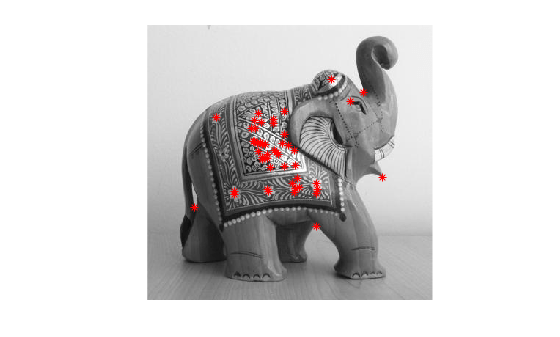keyPointsToStruct

Convert OpenCV KeyPoints object to MATLAB structure

Description

example

mlstruct = keyPointsToStruct(keypoints) converts an OpenCV KeyPoints object to a MATLAB® structure.

Examples

collapse all

This example shows how to use the prebuilt MATLAB interface for the OpenCV function cv::Fast in MATLAB to detect keypoints in an image. Additionally, use the keyPointsToStruct utility function to write the keypoints returned by the OpenCV cv::Fast function to a MATLAB structure.

Add the MATLAB interface to OpenCV package names to the import list.

import clib.opencv.*;
import vision.opencv.util.*;

Read an image into the MATLAB workspace.

Create MATLAB interface objects for the OpenCV MatND and InputArray classes to store the input image.

[inputMat,inputArray] = createMat(img);

Create a MATLAB interface object for the OpenCV KeyPoint vector by using the clibArray function.

keyPointsVec = clibArray("clib.opencv.cv.KeyPoint",0);

Specify the parameters for computing keypoints using the FAST detector.

threshold = 100;
nonmaxSuppression = true;

Compute keypoints in the image by calling the OpenCV function cv::FAST in MATLAB.

cv.FAST(inputArray,keyPointsVec,threshold,nonmaxSuppression);

Convert the KeyPoints object returned by the OpenCV function into a MATLAB structure.

mlstruct = keyPointsToStruct(keyPointsVec);

Inspect the fields in the output MATLAB structure.

mlstruct
mlstruct = struct with fields:
Location: [48x2 double]
Scale: [48x1 double]
Metric: [48x1 double]
Misc: [48x1 double]
Orientation: [48x1 double]

Display the input image and plot the detected keypoints.

figure
imshow(img)
hold on
plot(mlstruct.Location(:,1),mlstruct.Location(:,2),"*r")
hold offInput Arguments

collapse all

OpenCV KeyPoints class, specified as a MATLAB interface object. This interface object is a representation of the KeyPoints class returned by any of the OpenCV functions for keypoint detection.

Output Arguments

collapse all

Keypoints detected using the OpenCV function, returned as a MATLAB structure with fields Location, Scale, Metric, Misc, and Orientation.

 Fields Description Location x and y-coordinates of the keypoints. Scale Diameter of the neighborhood region around the keypoints. Metric Strength of the keypoints. Orientation Orientation of the keypoints.